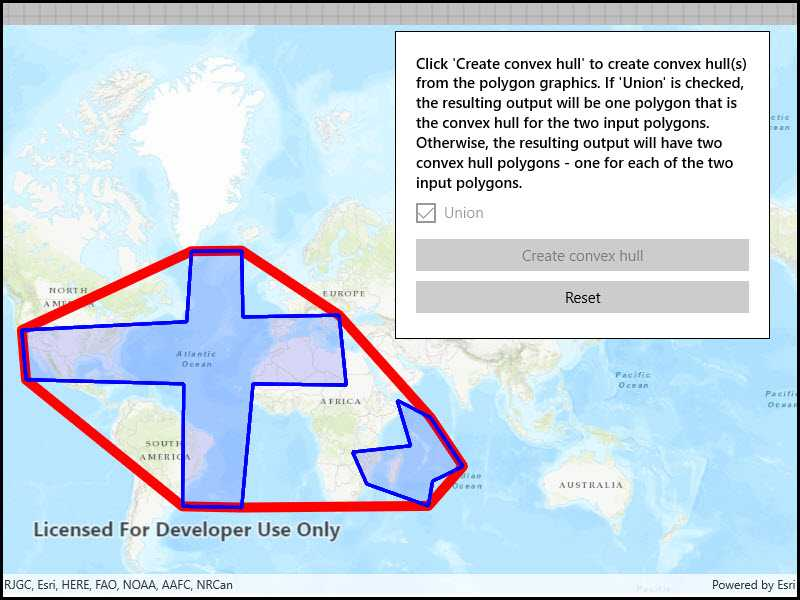# Convex hull list

Generate convex hull polygon(s) from multiple input geometries.## Use case

Creating a convex hull allows for analysis to define the polygon with the least possible perimeter that encloses a group of geometric shapes. As a visual analogy, consider a set of nails in a board where the convex hull is a rubber band stretched around the outermost nails.

## How to use the sample

Click the 'Create Convex Hull' button to create convex hull(s) from the polygon graphics. If the 'Union' checkbox is checked, the resulting output will be one polygon being the convex hull for the two input polygons. If the 'Union' checkbox is un-checked, the resulting output will have two convex hull polygons - one for each of the two input polygons. Click the 'Reset' button to start over.

## How it works

1. Create an `Map` and display it in a `MapView`.
2. Create two input polygon graphics and add them to a `GraphicsOverlay`.
3. Call `GeometryEngine.ConvexHull(inputGeometries, boolean)`, specifying a list of geometries for which to generate the convex hull. Set the boolean parameter to `true` to generate a convex hull for the union of the geometries. Set it to `false` to create a convex hull for each individual geometry.
4. Loop through the returned geometries and add them as graphics for display on the map.

## Relevant API

• GeometryEngine.ConvexHull
• Graphic.ZIndex
• GraphicsOverlay

## Tags

analysis, geometry, outline, perimeter, union

## Sample Code

ConvexHullList.xamlConvexHullList.xamlConvexHullList.xaml.cs
Use dark colors for code blocksCopy

``````﻿<UserControl
x:Class="ArcGIS.UWP.Samples.ConvexHullList.ConvexHullList"
xmlns="http://schemas.microsoft.com/winfx/2006/xaml/presentation"
xmlns:x="http://schemas.microsoft.com/winfx/2006/xaml"
xmlns:esriUI="using:Esri.ArcGISRuntime.UI.Controls">
<Grid>
<esriUI:MapView x:Name="MyMapView" />
<Border Style="{StaticResource BorderStyle}">
<StackPanel>
<TextBlock TextWrapping="Wrap"
FontWeight="SemiBold">
Click 'Create convex hull' to create convex hull(s) from the polygon
graphics. If 'Union' is checked, the resulting output will
be one polygon that is the convex hull for the two input polygons.
Otherwise, the resulting output will have two convex
hull polygons - one for each of the two input polygons.
</TextBlock>
<CheckBox x:Name="ConvexHullListCheckBox"
Content="Union"
Margin="0,5,0,5"
IsChecked="True" />
<Button x:Name="ConvexHullListButton"
Content="Create convex hull"
HorizontalAlignment="Stretch"
Margin="0,5,0,5"
Click="ConvexHullListButton_Click" />
<Button x:Name="ResetButton"
Content="Reset"
HorizontalAlignment="Stretch"
Margin="0,5,0,5"
Click="ResetButton_Click" />
</StackPanel>
</Border>
</Grid>
</UserControl>``````